Next: 6.6 Comparison with a Up: 6. Simulation of Thermal Previous: 6.4 Sharp Interface and

Subsections

# 6.5 Model Calibration

The simulated oxide thickness after a certain oxidation time must agree with the real physical thickness under the same assumed process conditions. The goal was to find a universal, but not complicated calibration method which works for all possible oxidation conditions, as described in the following.

## 6.5.1 Calibration and Parameter Extraction

A look to the model (see Section 3.2.1) shows that there are three available parameters, namely the diffusion coefficient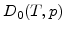, the maximal possible strength of the spatial sink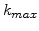, and the oxidant concentration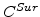at surfaces which have contact to the oxidizing atmosphere. As displayed in (3.9) and (7.1) the diffusion coefficient has a physical background. It is temperature and stress dependent and its real physical value can be determined correctly. Therefore to usefor calibration is not appropriate.

The next parameterhas more mathematical and modeling origin, but it is also not an optimal paramenter for calibration. At first the thickness of the reaction layer changes with, because it is inversely proportional to(see Section 3.2.4). This can be a problem for smallvalues which lead to thick reaction layers.

For a better understanding of the second trouble with largevalues the following is worth mentioning: Simulations have shown that with regard to the finite elements for the value ofthe following choice is reasonable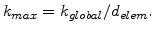(6.4)

Here,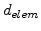is the average diameter of the finite elements in the used mesh, and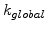is a constant value independent of the mesh fineness.

Due to the mesh dependence ofits variation is limited. In the experiments it was found out that the value ofcan not be increased arbitrarily. For larger values than suggested in (6.4) the numerical formulation becomes instable. In contrast to this, smallvalues are not a problem. Therefore,is not a suitable parameter for the model calibration of a potentially because of thick reaction layer (small value) and numerical instability (large value).

After excluding two of the three parameters, the last parameter which is the surface oxidant concentrationis investigated. On surfaces which have contact with the oxidizing atmosphere the oxidant concentration is used as a Dirichlet boundary condition. The key idea is to modifyin order to calibrate the oxide thickness of the simulated oxidation process over time for different oxidation conditions. From the physical aspect a higher surface oxidant concentration means that a larger number of oxidants diffuse to the Si/SiO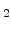-interface and react with silicon, which results in a faster oxidation rate.

## 6.5.2 Calibration Concept and Example

It was found with experiments that the best results are obtained ifconsists of a constant partand an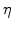-dependent part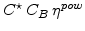so that the effective surface concentration can be written as a function of(6.5)

Here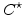is the standard oxidant concentration in the gas atmosphere as used in the Deal-Grove model.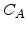,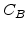, and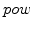are the calibration parameters. Because the value ofis changed during the oxidation process, the value ofis also changing with time. For the onward processgoes toward 0 on the surface and so the second term fordisappears.

As example for the above described calibration concept a (111) oriented and 0.4m height silicon block is wet oxidized and the oxide thickness over time for different temperatures is calibrated. The bottom surface is fixed, the lateral surfaces can only move vertically and on the upper surface a free mechanical boundary condition is applied. Only the upper surface of the body has contact with the oxidizing atmosphere. The oxide thickness is measured between the upper surface and the-level of 0.5.

In the calibration process the values of the parameters,, andare determined with the help of the in-house tool SIESTA (Simulation Environment for Semiconductor Technology Analysis) , so that the thickness values of the simulated oxide layers agree with the calculated physical reference values up to approximately 500nm at any time for a temperature range of 900-1100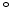C. The temperature dependent diffusion coefficient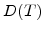is calculated as explained in (3.13). The other two model parameter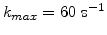and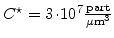are kept constant over the whole temperature range.

It was found that in case of wet oxidation the value of the parameter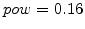in (6.5) can be hold constant for the temperature range of T=900-1100C. Furthermore, the experiments show that the parametercan be brought to a linear and the parametercan be brought to a parabolic dependence on temperature, which is described by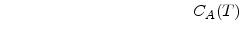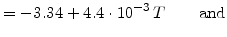(6.6)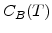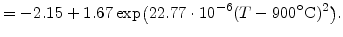(6.7)

The expressions forandwere found empirically. Their values over temperature are plotted in Fig. 6.11.anddo not have a physical background, they are pure fitting parameters.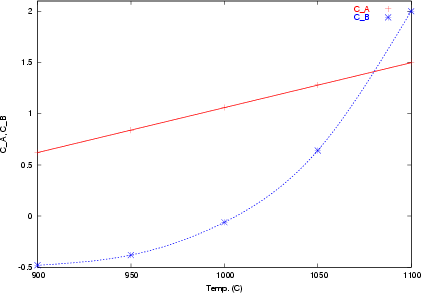In case of wet oxidation the three Figs. 6.12-6.14 show vicegerent for all other temperatures that the formula forwith its parameters,, andleads to an excellent agreement between the calculated reference curves and the measured simulation curves. The oxide thickness values for the reference curves are calculated with the Deal-coefficients . The coefficients,, andcan be also found without problems for other process conditions (e.g. dry oxidation), and so the calibration withalways works well.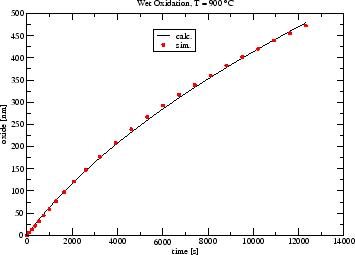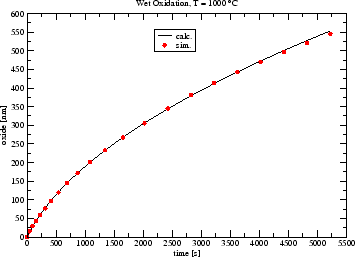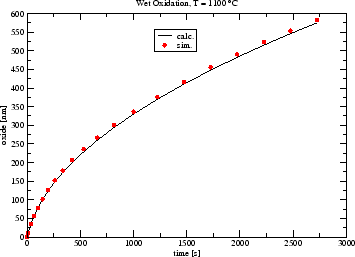Next: 6.6 Comparison with a Up: 6. Simulation of Thermal Previous: 6.4 Sharp Interface and

Ch. Hollauer: Modeling of Thermal Oxidation and Stress Effects